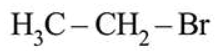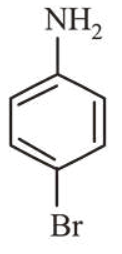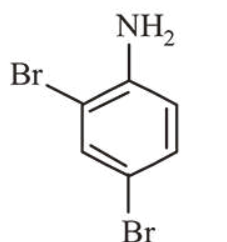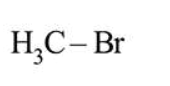# In Carius method of estimation of halogen,

Question:

In Carius method of estimation of halogen, $0.172 \mathrm{~g}$ of an organic compound showed presence of $0.08 \mathrm{~g}$ of bromine. Which of these is the correct structure of the compound?

1.2.3.4.Correct Option: , 2

Solution:

Mole of bromine $=\frac{0.08}{80}=10^{-3} \mathrm{~mole}$

Molar mass of compound is given by the following equation,

$\frac{0.172}{M}=10^{-3}$

$\Rightarrow M=\frac{0.172}{10^{-2}}=172 \mathrm{~g}$

$\because$ Molar mass of $\mathrm{C}_{6} \mathrm{H}_{6} \mathrm{NBr}$

$=(6 \times 12)+(1 \times 6)+(1 \times 14)+(1 \times 80)=172 \mathrm{~g}$

Thus option (b) is the correct structure of compound.